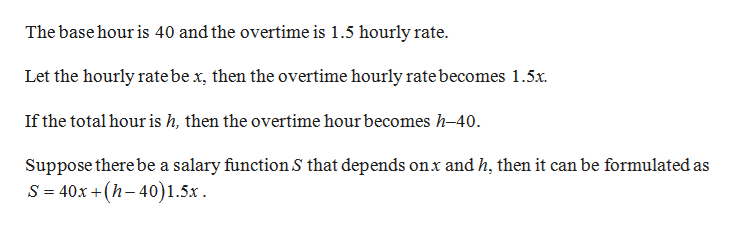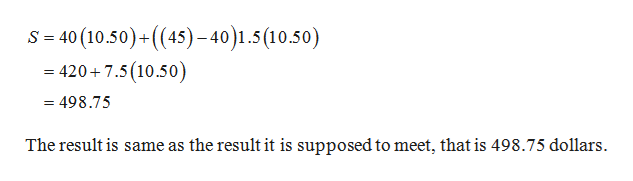# This has to be with python programming. I have to determine hoursworked using integer division and determine overtime hours using remainder division. Base hour is 40 and overtime is 1.5 hourly rate. How would I write the equation. Hint: if I plug in 45 hours and 10.50 rate per hour, I am suppose to get \$498.75. How did they come up with that solution?

Question
1 views

This has to be with python programming. I have to determine hoursworked using integer division and determine overtime hours using remainder division. Base hour is 40 and overtime is 1.5 hourly rate. How would I write the equation. Hint: if I plug in 45 hours and 10.50 rate per hour, I am suppose to get \$498.75. How did they come up with that solution?

check_circle

Step 1

Consider the gross pay or salary as the overtime hourly rate multiplied by the number of extra hours worked along with the regular pay, the hourly rate multiplied by the number of hours worked.help_outlineImage TranscriptioncloseThe base hour is 40 and the overtime is 1.5 hourly rate Let the hourly rate be x, then the overtime hourly rate becomes 1.5x If the total hour is h, then the overtime hour becomes h-40. Suppose there be a salary functionS that depends onx andh, then it can be formulated as S 40x +(h-40)1.5x fullscreen
Step 2

Verify the function by substituting the values h =...help_outlineImage Transcriptioncloses 40 (10.50) ((45)-40)1.5(10.50) = - 420 7.5(10.50) - 498.75 The result is same as the result it is supposed to meet, that is 498.75 dollars. fullscreen

### Want to see the full answer?

See Solution

#### Want to see this answer and more?

Solutions are written by subject experts who are available 24/7. Questions are typically answered within 1 hour.*

See Solution
*Response times may vary by subject and question.
Tagged in

### Math